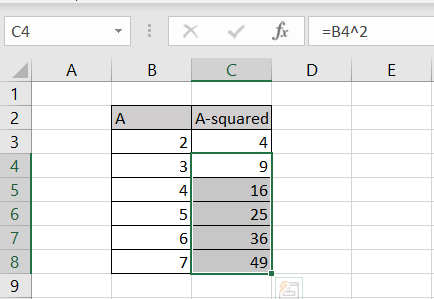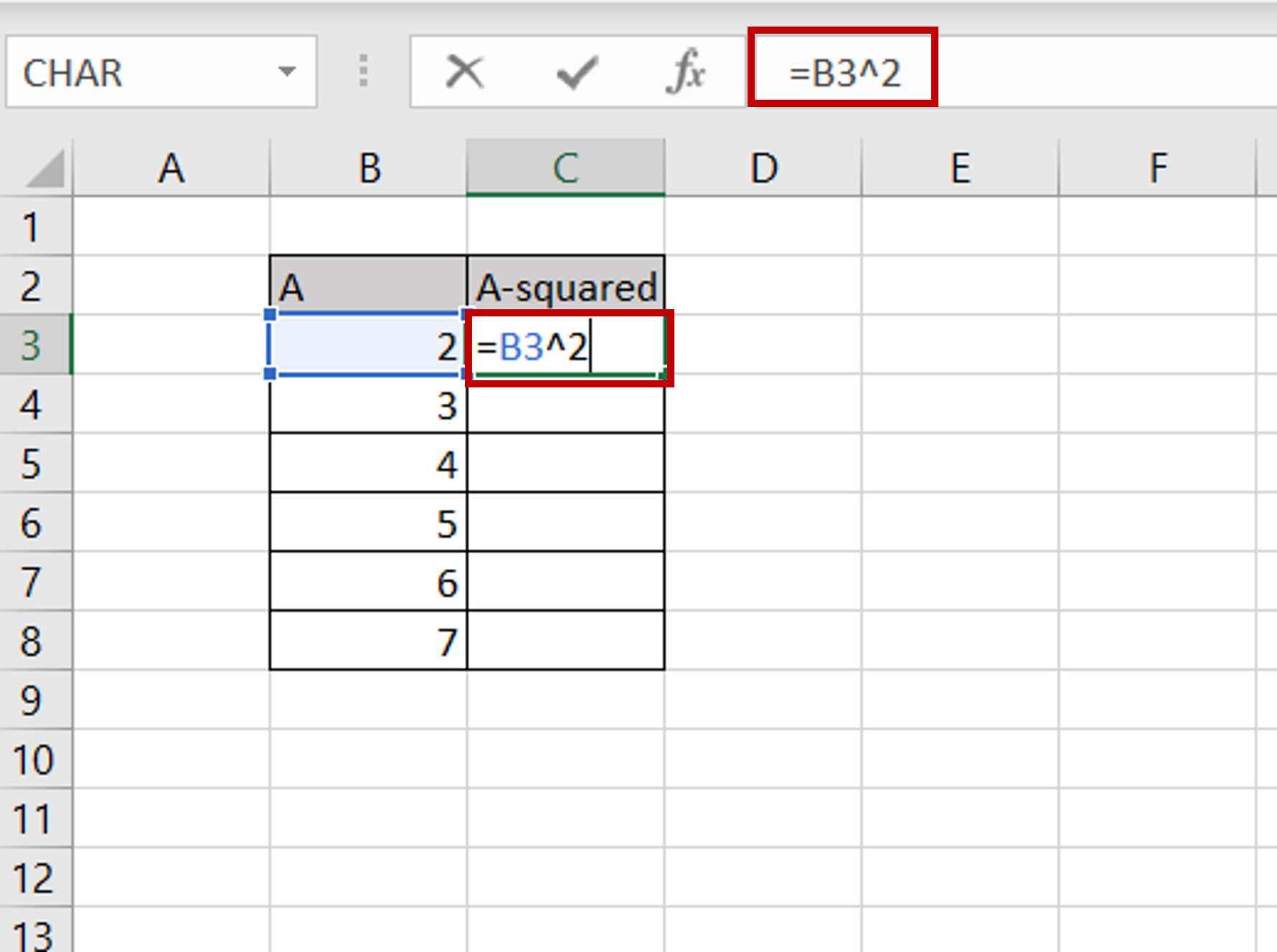# How to do squared on Excel

You can watch a video tutorial here.Excel is a great tool for calculation and provides many functions for mathematical operations and supports all basic mathematical operations. When performing calculations, you may need to square a number or a range of numbers. The caret (^) is used to square a number in Excel

### Step 1 – Create the formula-Select the destination cell
– Type the formula using cell references:
=A^2

### Step 2 – Copy the formula– Using the fill handle from the first cell, drag the formula to the remaining cells
OR
a) Select the cell with the formula and press Ctrl+C or choose Copy from the context menu (right-click)
b) Select the rest of the cells in the column and press Ctrl+V or choose Paste from the context menu (right-click)